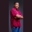Related Tags

range
set
swift
communitycreator

# How to check if a set of numbers is a subset of a range in SwiftTheodore Kelechukwu Onyejiaku

### Overview

A set stores elements or values that are unique. We can create a set of prime numbers and check if they are a subset of a particular range. A range is a series of values between a lower and an upper bound. When we specify a range, the lower bound is included within the range but the upper bound is excluded. For example, a range of 0–5 includes 0, 1, 2, 3, and 4.

To check if a set is a subset of a range, we use the isSubset(of:) method of the set class.

### Syntax

set.isSubset(of: range)
Syntax to check if a set is subset of a range

### Parameters

range: This is the range of values we specify.

### Return value

The value returned is a Boolean. A true will be returned if the set is actually a subset. Otherwise, a false will be returned.

### Code example

// create some set instances and initialize
let evenNumbers : Set = [2, 4, 6, 8]
let primeNumbers : Set = [2, 3, 5, 7]

// check if they are subset of a range
print(evenNumbers.isSubset(of: 0 ..< 10)) // true
print(primeNumbers.isSubset(of: 0 ..< 5))  // false
Check if a set is a subset of a range in Swift

### Explanation

• Lines 2–3: We create some set instances and initialize them with some values.
• Lines 6–7: We use the isSubset(of:) method to check if the set evenNumbers, which contains even numbers from 2 to 8, is a subset of the range of numbers from 0 to 10, and print the result.
• Line 7: Using the same isSubset(of:) method, we check if primeNumbers, which is a set of prime numbers from 2–7, is a subset of the range of values from 0 to 5, and print the result to the console.

RELATED TAGS

range
set
swift
communitycreator

CONTRIBUTORTheodore Kelechukwu Onyejiaku
RELATED COURSES

View all Courses

Keep Exploring

Learn in-demand tech skills in half the time